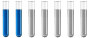Nernst equation

Chemistry and homework help forum.

Organic Chemistry, Analytical Chemistry, Biochemistry, Physical Chemistry, Computational Chemistry, Theoretical Chemistry, High School Chemistry, Colledge Chemistry and University Chemistry Forum.

Share your chemistry ideas, discuss chemical problems, ask for help with scientific chemistry questions, inspire others by your chemistry vision!

Please feel free to start a scientific chemistry discussion here!

Discuss chemistry homework problems with experts!

Ask for help with chemical questions and help others with your chemistry knowledge!

Moderators: expert, ChenBeier, Xen

Neon
Jr. MemberPosts: 6
Joined: Fri Jun 15, 2018 12:11 pm

Nernst equation

Hello,

I would like to ask you a question about Nernst equation. So this is the correct notation of Nernst's equation if I want to calculate the pH measured with a pH meter?:
$$E= E0 + (2,3RT)/(nF) * pH$$
So does a pH meter use this equation to calculate pH?